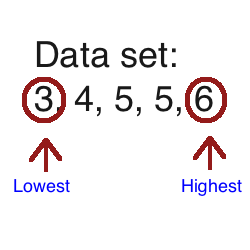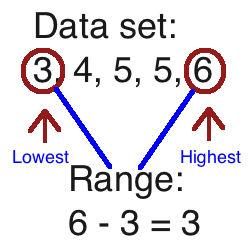What is Range in Math? - Lesson for Kids

Instructor: Trisha Fyfe

Trisha has taught college and K-12 English, reading, writing, and math. She has a master's degree in teaching.

In this lesson, you'll learn the definition of range. You'll learn how to find the range of a group of numbers using examples, and then you can test your new skill with a short quiz.

What Is Range?

A group of five students in your class decided to have a race, and it's up to you to find difference between the slowest and fastest times. To identify this, we need to find the range in this data set.

A data set is a group of numbers that has been collected. The range is the difference between the lowest and highest numbers in a data set. Essentially, the range tells us how spread apart a group of numbers is.

How Do I Find Range?

Finding the range for a group of numbers is easy. Let's go through the steps and find the range for the student race.

Step 1: Identify the Data Set

We first need to identify our data set. In this scenario, the data set is a list of times (minutes) it took for each of the 5 students to run around the track. Let's say their times were: 5, 3, 4, 5, and 6 minutes. Our data set is: 5, 3, 4, 5, 6.

Step 2: Arrange Numbers

Next, list the numbers in order from lowest to highest. So, for our race times, we arrange the numbers in this order:As you can see, this makes it much easier to find the lowest number (3) and highest number (6). When we are finding the range, we don't use the numbers in between at all!

Step 3: Do the Math

To calculate the range, subtract the lowest number from the highest number. If the lowest number is 3 and the highest number is 6, we solve for 6 - 3. Since the answer is 3, our range for this data set is 3.So how do we use this information? The range can tell you how spread apart a group of numbers are. So in our example, if the range in the students' race times was three minutes, that means there was a 3-minute difference between the slowest and fastest runner.

To unlock this lesson you must be a Study.com Member.

Register to view this lesson

Are you a student or a teacher?

See for yourself why 30 million people use Study.com

Become a Study.com member and start learning now.
Back
What teachers are saying about Study.com

Earning College Credit

Did you know… We have over 200 college courses that prepare you to earn credit by exam that is accepted by over 1,500 colleges and universities. You can test out of the first two years of college and save thousands off your degree. Anyone can earn credit-by-exam regardless of age or education level.